The perfect place for easy learning...

×

# C Input Functions

C programming language provides built-in functions to perform input operations. The input operations are used to read user values (input) from the keyboard. The c programming language provides the following built-in input functions.

1. scanf()
2. getchar()
3. getch()
4. gets()
5. fscanf()

# scanf() function

The scanf() function is used to read multiple data values of different data types from the keyboard. The scanf() function is built-in function defined in a header file called "stdio.h". When we want to use scanf() function in our program, we need to include the respective header file (stdio.h) using #include statement. The scanf() function has the following syntax...

# Example Program

``````#include<stdio.h>
#include<conio.h>

void main(){
int i;
printf("\nEnter any integer value: ");
scanf("%d",&i);
printf("\nYou have entered %d number",i);

}```
```

Output: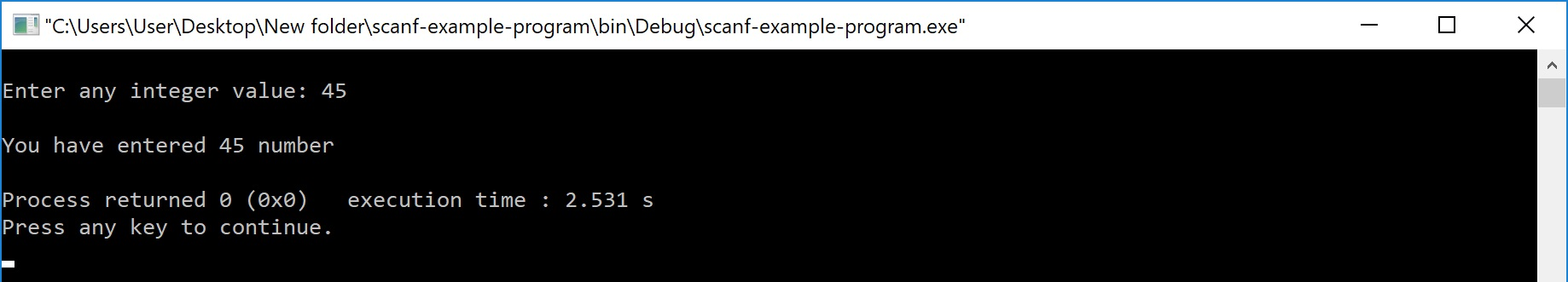In the above example program, we used the scanf() function to read an integer value from the keyboard and store it into variable 'i'.

The scanf function also used to read multiple data values of different or the same data types. Consider the following example program...

# Example Program

``````#include<stdio.h>
#include<conio.h>

void main(){
int i;
float x;
printf("\nEnter one integer followed by one float value : ");
scanf("%d%f",&i, &x);
printf("\ninteger = %d, float = %f",i, x);

}```
```

Output: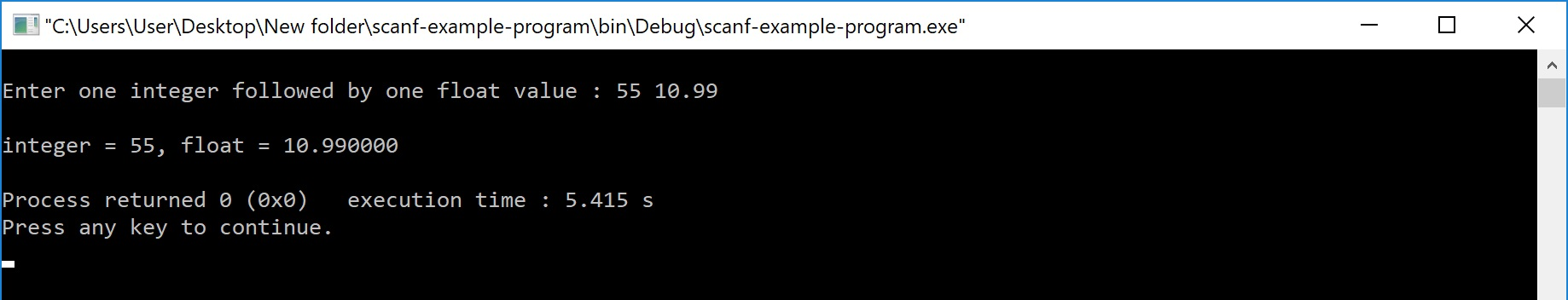In the above example program, we used the scanf() function to read one integer value and one float value from the keyboard. Here 'i' is an integer variable so we have used format string %d, and 'x' is a float variable so we have used format string %f.

The scanf() function returns an integer value equal to the total number of input values read using scanf function.

# Example Program

``````#include<stdio.h>
#include<conio.h>

void main(){
int i,a,b;
float x;
printf("\nEnter two integers and one float : ");
i = scanf("%d%d%f",&a, &b, &x);

}```
```

Output: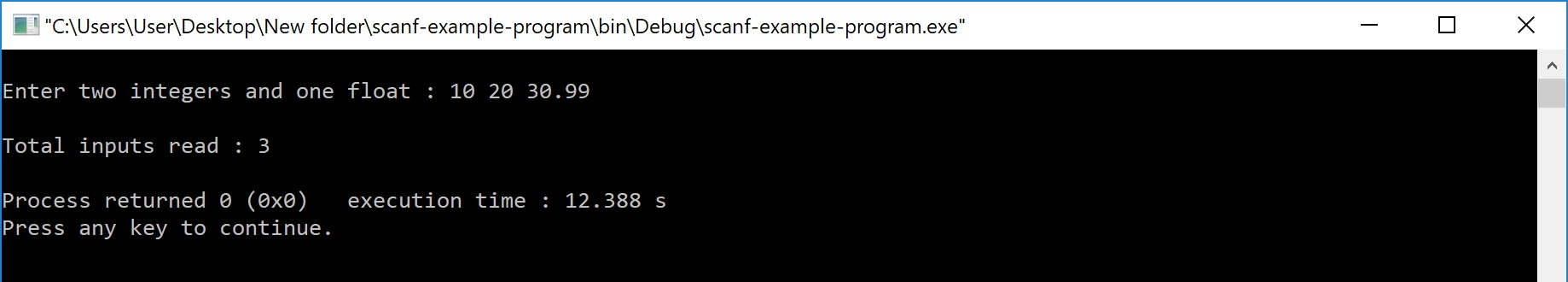# getchar() function

The getchar() function is used to read a character from the keyboard and return it to the program. This function is used to read a single character. To read multiple characters we need to write multiple times or use a looping statement. Consider the following example program...

# Example Program

``````#include<stdio.h>
#include<conio.h>

void main(){
char ch;
printf("\nEnter any character : ");
ch = getchar();
printf("\nYou have entered : %c\n",ch);

}```
```

Output: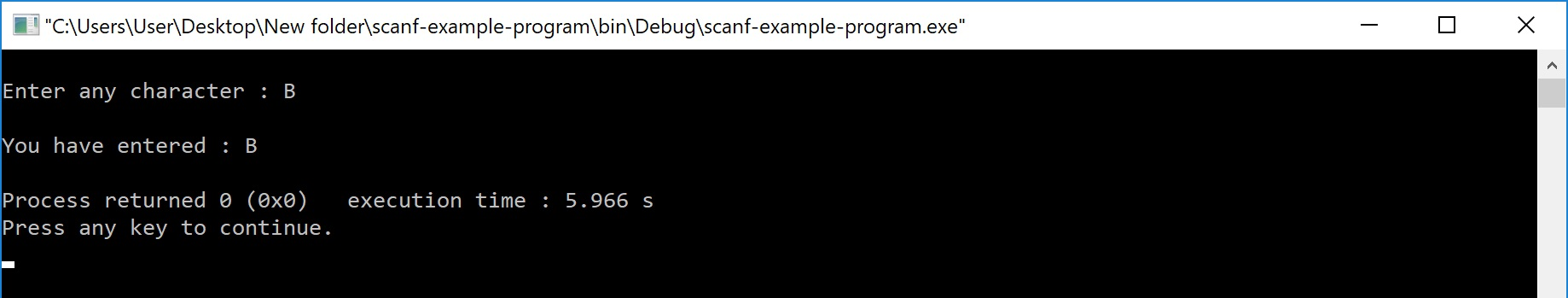# getch() function

The getch() function is similar to getchar function. The getch() function is used to read a character from the keyboard and return it to the program. This function is used to read a single character. To read multiple characters we need to write multiple times or use a looping statement. Consider the following example program...

# Example Program

``````#include<stdio.h>
#include<conio.h>

void main(){
char ch;
printf("\nEnter any character : ");
ch = getch();
printf("\nYou have entered : %c",ch);

}```
```

Output: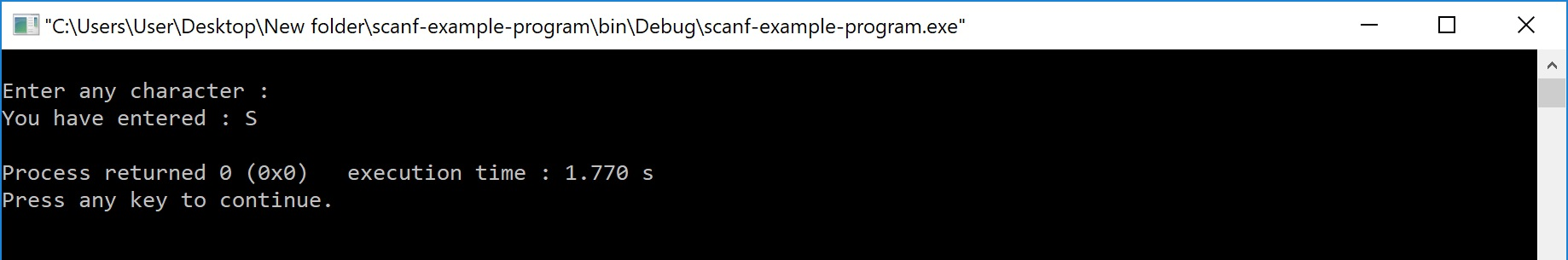# gets() function

The gets() function is used to read a line of string and stores it into a character array. The gets() function reads a line of string or sequence of characters till a newline symbol enters. Consider the following example program...

# Example Program

``````#include<stdio.h>
#include<conio.h>

void main(){
char name;
gets(name);
printf("%s",name);
}```
```

Output:# fscanf() function

The fscanf() function is used with the concept of files. The fscanf() function is used to read data values from a file. When you want to use fscanf() function the file must be opened in reading mode.